# How to Assess the Quality of Zeta Potential Results

The measurement of zeta potential is done by primarily measuring a quantity termed as electrophoretic mobility then converting that value by using electrokinetic theory. Mobility is described as the velocity that a particle would attain if a unit electrical field was applied.

In fact, an electric field, as high as is possible or desirable, is applied: the field and velocity are measured as exactly as possible and the electrophoretic mobility is obtained from the simple relationship:

Mobility = Velocity/Electric field

Zeta potential, and hence mobility, can be either negative or positive in sign, and hence the direction of travel is subject to the sense of the electric field.

The electric field can be calculated by either measuring the voltage across the measurement cell or from the sample’s conductivity and the electric current flowing when the field is applied. Actually, the second technique is used when the sample’s conductivity is high enough to make this exact, as it accounts for effective changes because of electrode polarization.

## The Zetasizer Nano

The electronics fitted to the Zetasizer Nano are able to make correct measurements of the applied field under all measurement settings so the end accuracy of the result relies on the ability to measure the velocity of the particle.

Velocity is measured by detecting the change in frequency of light dispersed by the particles, the famous ‘Doppler Effect’. In the Zetasizer Nano, the process of Phase Analysis Light Scattering is employed. This measures the frequency variation by comparing the relative phase of the scattered signal with that of a reference signal, and is highly sensitive so that small frequency shifts can be measured. In the Zetasizer Nano, this reference is produced by an optical modulator at a frequency of between 250 and 320 Hertz. This allows signals above and below the modulator to be identified and the electric field is applied so that higher frequencies match to positively charged particles and lower to negative.

By definition, phase = frequency x time, so measuring the variation in phase as a function of time enables the frequency shift and hence mobility to be established. The field is reversed intermittently with a period of between 26 and 45 milliseconds. These periods are fixed for different measurement conditions in various designs of a cell.

Since frequency shift is proportional to mobility x electric field, it would appear that in order to establish a small value of mobility, you could just increase the field to higher values. In reality, there are limitations because of the conductivity of numerous types of sample. The likelihood of electrical breakdown even in non-conductive samples restricts the electric fields that can be used safely. It is better to use the ability of PALS to make correct measurements of small frequency variations than to increase the applied field.

So as to make a measurement, numerous reversals of the electric field are applied continually in a burst referred to as a ‘run’. The field reversal is to guarantee the net field in each direction is the same and to decrease any polarization effects such as the evolution of gas at the electrodes.

One run is nearly never enough as the particles are in random motion (Brownian motion). There are also systematic fluid flows because of convection and the additional (Joule) heating effect of transmitting an electric current through a conductive medium. The result of all these other effects is to incorporate random phase changes to the systematic changes produced by the electrophoretic mobility of the sample. The mobility effects are synchronized with the variations in the direction of the applied field, while the thermal and other random motion effects are not. It is possible to incorporate runs together so that the noise effects are likely to cancel while the systematic electrophoretic effects reinforce.

The matter then is to plan how many runs to undertake to get an acceptable result, and to know how correct the result is based on calculations on the data itself. The measurement can then also be repeated on the same sample, and also various aliquots of the sample, to see that reliable results are attained.

## Phase Plot of a Standard DTS1050 Measurement

The phase plot of a measurement of the DTS1050 reference material is illustrated below. This is said to be an ‘easy’ sample as its mobility of -4 µm/sec/v/cm is fairly high. (Representative electrophoretic mobility values range from -10 to +10 expressed in these outdated but convenient units)

It can be observed from the figure, the regular saw-tooth waveform formed by the field switching, superimposed on a wavy background as a result of the thermal drifts is also present. In this case, the signal — the saw-tooth — is much larger than any higher frequency noise and easily differentiated from the slower background drift.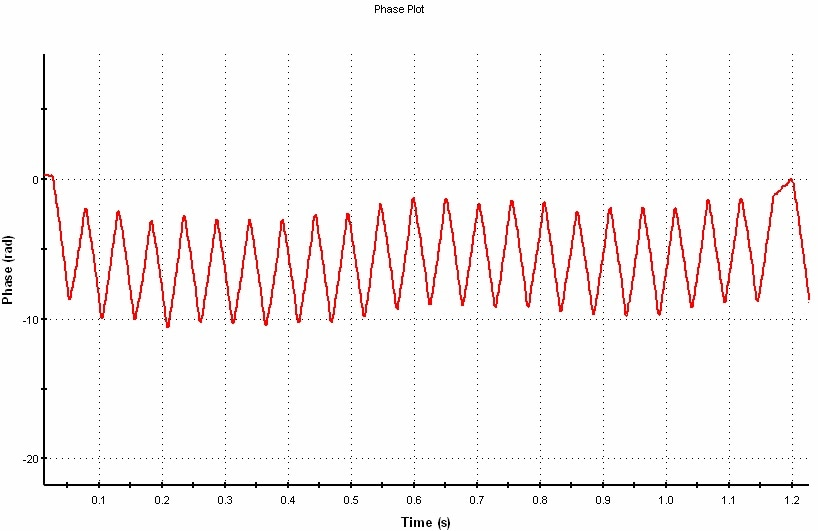Figure 1. Phase plot of a standard DTS1050 measurement

Figure 2 shows that the noise appears to be larger than any systematic electrophoretic signal. The data could perhaps be enhanced by performing a longer measurement, but what is required is some unbiased way of measuring the ratio of signal-to-noise in a phase plot so as to ‘know’ how long to continue measuring and then to evaluate how accurate the result is.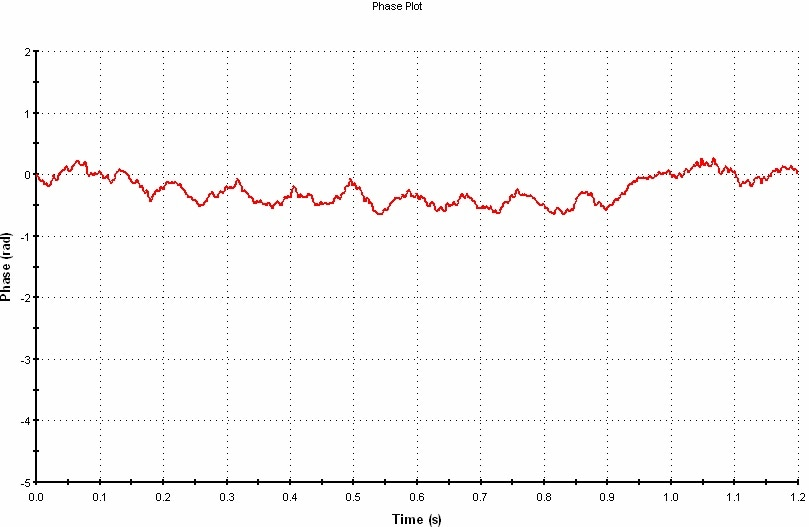Figure 2. Phase plot of a low mobility sample

In version 5.00 software, Micromeritics has combined the calculation of a quality factor designed to perform this function. Since the signal has a major component at the frequency of the applied field while the noise is estimated to be present at all frequencies, they compare the power in the phase spectrum at the field frequency with the average power at other frequencies. This is achieved internally by performing a Fourier Transform on the data and selecting the peak at the expected frequency arising from the electrophoretic effect.

Figure 3 illustrates an example of a more ‘tough’ sample in that it is a low mobility material. The mobility value of -0.1 makes it about 40 times more ‘difficult’ than the DTS1050 standard. After one run, just choose a peak at channel 18 which matches the applied field frequency. The area under this peak is compared to the noise level (signified by the error bars on the frequency data neighboring the peak) and the ratio calculated and scaled in such a way the ‘good’ data will have a value > 1.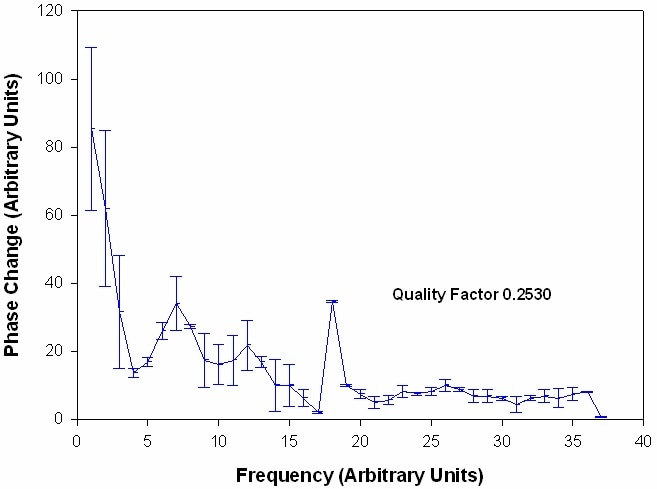Figure 3. Fourier transform of 1 run of phase data for a sample of mobility 0.1

The noise is estimated by changes in the spectrum from channel to channel. Here, the channels used to estimate the noise go from 10 to 16 and 20 to 35: the peak from 17 to 19. (This selection is based on the actual frequency of the field which can have various settings).

Note that at low frequencies, the noise error bars are about +/- 20 units, at higher frequencies +/- 5 or less compared to a peak height of 40. A quality factor of 0.25 was computed for this single run so a longer measurement is required (see Figure 3).

Figure 4 illustrates that after 150 runs, the quality factor has increased to 1.07 as seen in Figure 4. The peak currently reaches 30 units from a lower baseline while the noise levels as signified by the error bars on the spectrum are currently of the order +/- 1.5 below the peak and less than that at higher frequencies.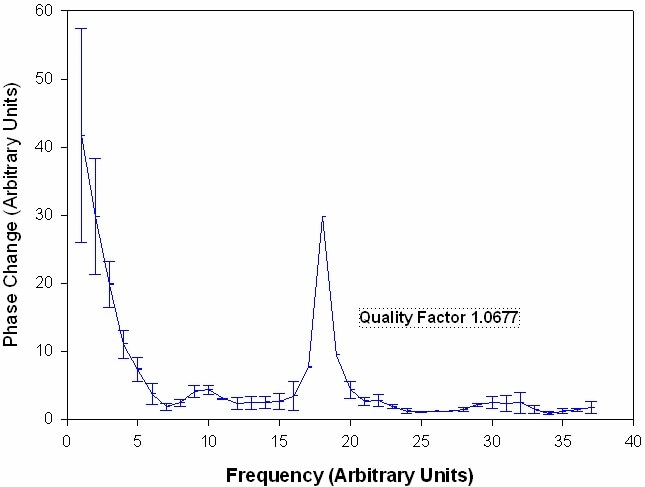Figure 4. The same measurement after 150 runs have been averaged

Figure 5 graphically shows how the quality factor differed during this measurement. In this case, the quality factor is plotted every 10 runs.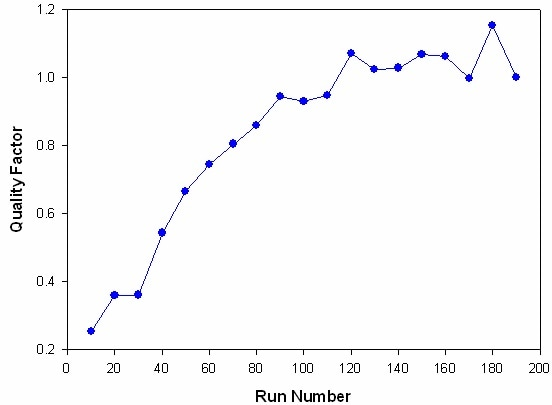Figure 5. Evolution of quality factor plotted against run number

Note that after around 120 runs, the quality is likely to level off and more runs will not create a marked improvement. This effect is to be expected as the measurement is not merely limited by random noise. The measurement process itself creates more noise because of the passage of electric current and possible degradation of sample and electrodes. Additional improvement might well necessitate much longer run times. In this case, as the target value of 1 is attained after about 120 runs, the automatic measurement duration will come to an end, providing the result stays stable.

For very low mobility samples, it is essential to perform a minimum number of measurements before the quality factor can be depended on. This is because random fluctuation in the phase spectrum will occur in the ‘peak’ as well as the areas to either side so spuriously ‘good’ values can be gained from a few runs.

The automatic measurement is usually arranged so that there is a minimum of 10 runs and a maximum of 100 (Figure 6). The measurement will thus stop sometime after 10 but before 100 runs if the quality factor of one has been reached. These run number settings are adjustable by the user. Shortening the automatic duration below the level set is not advised. It is obviously possible to perform a manual measurement of any number of runs. The quality factor can be exhibited in the record view or on another report as necessary. It is used by the ‘expert system’ to establish if adequate runs have been performed.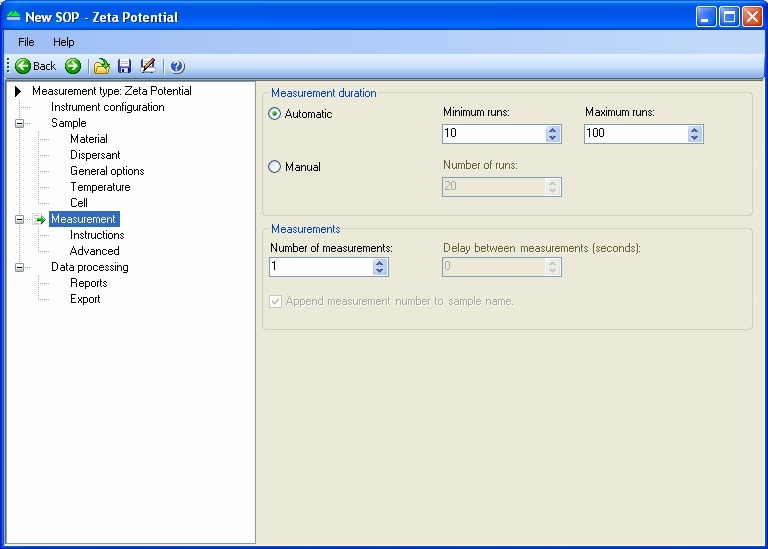Figure 6. Typical zeta potential measurement setup in the Nano software

## Conclusion

Having performed a measurement that obtains a good quality factor, how good is that measurement? To measure the mobility, the phase change as a function of time — that is the gradient of the saw-tooth segments in the phase plot is calculated. To achieve this, the separate field reversals throughout the run data are averaged and a linear fit performed. Besides calculating the gradient, the relative error in the gradient and also the correlation coefficient R that establishes if the phase data really are well denoted by a straight line, are calculated.

In Table 1, it can be observed that from a data fitting point of view, a good quality result will possibly have a mobility error of 0.01, i.e. +/- 1% of the mobility value. This looks like a good target to accomplish. It is then suggested that measurements are done on several samples, and the run variation established, which is likely to be larger than the ambiguity from the data analysis.

Table 1. Quality factor, mobility error and correlation coefficients for different mobility data

Quality Factor Relative Mobility Error Correlation Coefficient
2 to 5 0.01 0.999
1.0 0.015 0.995
0.5 0.02 0.992
50.25 0.08 0.957This information has been sourced, reviewed and adapted from materials provided by Micromeritics Instrument Corporation.

## Citations

• APA

Micromeritics Instrument Corporation. (2019, December 09). How to Assess the Quality of Zeta Potential Results. AZoM. Retrieved on October 27, 2020 from https://www.azom.com/article.aspx?ArticleID=16801.

• MLA

Micromeritics Instrument Corporation. "How to Assess the Quality of Zeta Potential Results". AZoM. 27 October 2020. <https://www.azom.com/article.aspx?ArticleID=16801>.

• Chicago

Micromeritics Instrument Corporation. "How to Assess the Quality of Zeta Potential Results". AZoM. https://www.azom.com/article.aspx?ArticleID=16801. (accessed October 27, 2020).

• Harvard

Micromeritics Instrument Corporation. 2019. How to Assess the Quality of Zeta Potential Results. AZoM, viewed 27 October 2020, https://www.azom.com/article.aspx?ArticleID=16801.